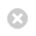Close

UCL Great Ormond Street Institute of Child Health

HomeGreat Ormond Street Institute of Child Health# Standard Error

### What a sample tells us

To illustrate the power of a random sample to give information about the population, consider the following example.

Example:

Shown below is the distribution of birthweights of 17,333 babies born consecutively in 3 West London hospitals. The mean birthweight was 3263.57g (7lb 3oz), standard deviation 551.71g (1lb 3oz):

This is the TOTAL POPULATION of birthweights in those hospitals in the chosen time period. The distribution of birthweights is clearly normal.

Suppose that we had wished to know the average birthweight in the area served by the hospitals over the relevant time period, but were only able to record the weights of 30 random births.

A random sample of 30 birthweights (chosen by computer) from the births at the hospitals is shown below:We can show this sample as a bar chart:This sample of 30 birthweights randomly selected from the 17,333 has a mean of 3379.0g and standard deviation 596.22g.

If we had taken only this sample from the population then our BEST GUESS of the average birthweight of that population is given by the MEAN OF THE SAMPLE (i.e., 3379g). This guess is unlikely to be completely accurate; we would not expect a sample of 30 to give us the exact mean of the total population of 17,333. However, we do have a better idea of the mean in the population after having sampled the 30 values.

If we repeat the process and take another sample of 30 babies then we will have another estimate of the population mean. The mean of the second sample is highly unlikely to be the same as that in the first sample. The means of the samples from repeating the process will vary. To illustrate the variability of sample means obtained by repeatedly taking samples of the same size (in this case 30 birthweights), the process was repeated 5 more times and the results are shown below.The sample means vary, sometimes over-estimating the population mean (for example, samples 1,3,4,5 and 6) and sometimes under-estimating (for example, sample 2). It is very unlikely that we would ever obtain the true population mean exactly from a sample of 30.

However, if we consider where the sample means lie on the distribution of population birthweights, we can see that the sample estimates are actually relatively near to the population mean:

A sample as small as 30 from this population of 17,333 is actually very informative in terms of giving us information re the mean of the population. The sample means cluster around the true 'population' mean and are just as likely to over as to under-estimate the population mean.

If we continued to take samples of size 30, we could form a distribution of the sample means that we obtain. This distribution would be normally distributed around the population mean and less spread than the actual values.

### Standard error

A general result is that if the population has a mean µ and standard deviation σ, then the sample means of samples of size n will be normally distributed with mean µ and standard deviationThe standard deviation of the sample means is known as the standard error. This is a measure of how precisely any one sample is likely to estimate the population mean.

NOTE that the standard deviation describes the spread of a sample; standard error is a measure of the precision with which the sample statistic approximates the true population value. The standard error must, by definition, become smaller as the sample size increases.Larger samples will give a smaller standard error and hence a less spread out distribution. The less spread the distribution, the closer any one sample mean is likely to be to the population mean.

The STANDARD ERROR is the name given to the precision with which a sample estimate approximates the population value. The standard error is not an estimate of any quantity in the population, but is a measure of the uncertainty of a single sample value as an estimate of the population value.

In real life situations we would only have one estimate of the population mean from our single random sample.

We do not usually know the population standard deviation, so we estimate it using the standard deviation of the sample. The estimate from the sample can be assumed to be ok if:

• The sample is at least approximately normally distributed and
• The sample consists of at least 20 measurements.

Even when we have a large sample that is approximately normally distributed we know that our estimate is still unlikely to be exactly correct. However, any error in the sample estimate of the population standard deviation will be reduced when it is divided by the square root of the sample size in construction of the standard error estimate. If the sample is large, then the erroneous value is divided by a relatively large number and any error will be diminished further than if a small sample were used. Hence, there is a trade-off between the degree of non-normality that can be tolerated before the sample estimate becomes too inaccurate and the size of the sample.

For example:

The 6 random samples of 30 birthweights selected by the computer had standard deviations 596.17, 527.11, 520.72, 550.91, 569.87 and 543.18, which are up to 44.46g away from the true value of the population standard deviation (551.71g).

These 6 samples, based on 30 measurements each, lead to standard error estimates of 108.85, 96.24, 95.07, 100.59, 104.05 and 99.17 respectively, all within 8.12g of the true value.

Hence the errors in the standard error estimates are smaller than the errors in the standard deviation estimates. If the sample used had been larger than the standard deviation estimates would have been divided by a larger quantity and the errors in the standard errors reduced further.